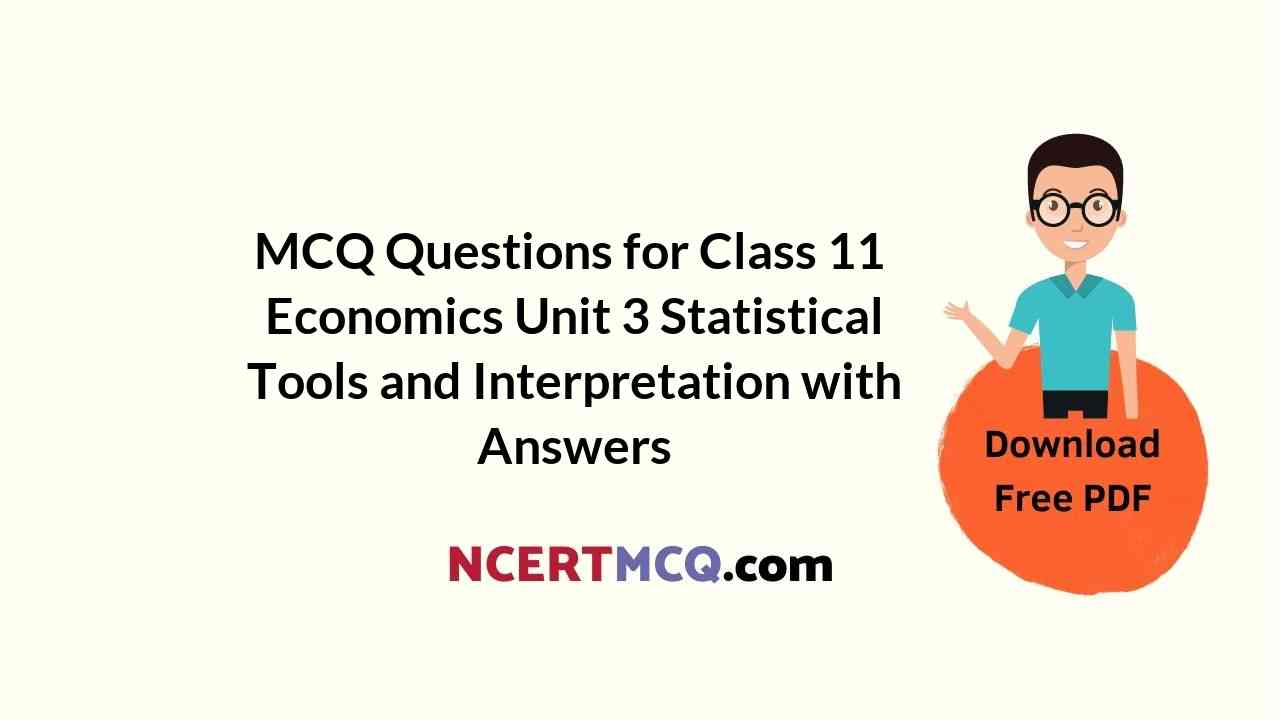Check the below NCERT MCQ Questions for Class 11 Economics Unit 3 Statistical Tools and Interpretation with Answers Pdf free download. MCQ Questions for Class 11 Economics with Answers were prepared based on the latest exam pattern. We have provided Statistical Tools and Interpretation Class 11 Economics MCQs Questions with Answers to help students understand the concept very well.

## Statistical Tools and Interpretation Class 11 MCQs Questions with Answers

MCQ Questions For Class 11 Statistics Economics Chapter 3 Question 1.
The most common measurement of central tendency is:
(a) Median
(b) Multiple
(c) Mean
(d) Weighted mean.

Class 11 Statistics Chapter 3 MCQ Questions Question 2.
Division of one column in ten equal parts is called:
(a) Decile
(b) Quartile
(c) Percentile
(d) None.

Statistics For Economics Class 11 Chapter 3 MCQ Questions And Answers Question 3.
Which of the following equation is correct:
(a) Anova = σ
(b) Anova = σ-2
(c) Anova = σ4
(d) Anova = √σ × 2.

Statistical Tools And Interpretation Class 11 MCQ Question 4.
Where is correlation multiple placed:
(a) Between 0 and + 1
(b) Between – 1 and 0
(c) Between – 1 and + 1
(d) None of these.

Answer: (c) Between – 1 and + 1

Ch 3 Statistics Class 11 MCQ Question 5.
Base year is:
(a) Comparison year
(b) Present year
(c) Any year
(d) One year previous to present year.

Fill in the blanks:

1. Rule of ……………………….. is supposed to be idle for creation of index.

2. Multiple is the value of column in which ……………………… comes repeatedly.

3. The value which divides the column into more than two parts is called ……………………………

4. ………………………. is the difference between maximum and minimum value.

5. …………………………. is the simple ray that shows relation between two values.

State true or false:

1. Cumulative frequency shows “less than” or “more than” of any sequence.

2. 5 is the mode of the numbers 3, 4, 3, 5, 5, 3, 2.

3. Range is found by adding highest value and smallest value.

4. Money inflation is measured on changes of index number of whole sale market of weekly statement.

5. High income leads high savings.

Match the columns:

 Column A Column B 1. $$\frac { Σx }{ N }$$, here Σx is (a) Positive or negative 2. Formula of range (b) Minimum effect of values 3. Type of correlation (c) Total of all values 4. Consumer Price Index (d) $$\frac { H-L }{ H+L }$$ 5. Median (e) Cost of living index.

 Column A Column B 1. $$\frac { Σx }{ N }$$, here Σx is (c) Total of all values 2. Formula of range (d) $$\frac { H-L }{ H+L }$$ 3. Type of correlation (a) Positive or negative 4. Consumer Price Index (e) Cost of living index. 5. Median (b) Minimum effect of values

1. Graphic method of studying dispersion.

2. Definite relation between two or more groups.

3. One which divides column in ten equal parts.

4. In India which index helps to measure average change in prices.

5. Saving is directly related to.

6. What is measure of central tendency?

Answer: Measure of central tendency is the single value which represents the characteristics of the entire universe.

7. Which is the popular measure of central tendency?

8. Define Arithmetic mean?

Answer: It is defined as the sum of the values of all observations divided by the number of observations.

9. Find out mode from the following data:
7, 12, 8, 5, 9, 10, 9, 11, 96

Answer: Weighted Arithmetic Mean = $$\frac{ΣWx}{ΣW}$$